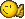# Find yaw/pitch of one vector relative to another

## Recommended Posts

This is a 3D problem that I am trying to solve. I have a scenario where I need to be able to travel in a direction (v) while maintaining a line of sight with a point in 3D space (u). However, one restriction is that the line of sight must be confined to a fixed viewing area. For instance, the camera traveling in the v direction is restricted so that it can only tilt up/down 45 degrees and turn left/right 50 degrees. If the point in 3D space is too far behind the camera, then the camera will no longer be able to point in the precise direction of the point, but it would point in the general direction of the point.

I've been tackling this problem for some time now, and conceptually, it is very easy to understand. The most confusing aspect to me is how to accurately calculate the angles for the yaw and pitch.

What I've done so far....

To determine the values for the camera's direction vector, I am taking the known yaw and pitch angles (my current heading) and calculating the x, y, z coordinates onto a unit sphere.

v.x = cos(alpha) * cos(beta);

v.y = sin(beta);

v.z = sin(alpha) * cos(beta);

This produces my direction vector in an already normalized form. I then take the point in space that I'm looking at and subtract my current position to create the vector, u, to the point in 3D space. I am not normalizing this vector.

Once I've created these two vectors, I attempt to get the yaw and pitch angles between them. To do this, I create a new vector, w, by subtracting u from v (so w = v - u). I then normalize that vector. I know that the yaw angle will be the asin of the y component of the w vector.

yaw = asin(w.y)

That yaw value can then be plugged in to my sphere equation to give pitch. Of course, I'll have to account for situations where cos(yaw) equals 0.

pitch = acos(w.x / cos(yaw))

However, the results that I'm seeing don't match what I am expecting to see. As an example... in some cases, a yaw angle of 82 might be calculated when I'm actually expecting 98. I know that this is due to the fact that my C compiler returns asin values in the range -PI/2 to PI/2, however I don't know how to deal with it.

Incidentally, I'm not as concerned with verifying whether or not the camera can actually position itself to view the point. That will be relatively easy. I included that in the description to help indicate *why* I need to know the exact values of the pitch and yaw.

Also, the roll angle at this point is 0, but I can't guarantee that it will always be so.

Does anyone have any experience with this type of problem? If so, any help would be greatly appreciated.

Thanks

##### Share on other sites

However, the results that I'm seeing don't match what I am expecting to see. As an example... in some cases, a yaw angle of 82 might be calculated when I'm actually expecting 98. I know that this is due to the fact that my C compiler returns asin values in the range -PI/2 to PI/2, however I don't know how to deal with it.

82 and 98 is too big difference and in no way can be connected with certain C amth library implementation.

The most confusing aspect to me is how to accurately calculate the angles for the yaw and pitch.

I have read through your problem, however I didn't have time to write it out to understand your approach in details, so I wrote how I would find the angles.

Here is how I see it.

If I'm assuming correctly, yaw is horizontal rotation (looking left/right) and pitch is looking up and down (English isn't my 1st language). I assume you don't have a roll component (like in most fps).

The second assumption is that you want angles relative to the direction of v (in fps it is always parallel to z-axis)

Yaw is simple. You have moving direction vector v and viewing direction u. To get yaw angle, project 2 vectors on z-plane, i.e. just taking the same vectors with z=0, then just find angle between 2 resulting vectors. Yaw angle is relative to v.

Pitch is a bit complicated when . You have to project u on the plane of v. Assuming you have same turning model as in first person shooters, it is the plane, such that v belongs to that plane and every vector that belongs to the same plane and perpendicular to v is parallel to xy-plane (tada!). Take vector v and the other vector (say x) that meets above requirements and take equation of the plane, then project u on resulting plane. After that get angle between u_proj and u. It is your pitch angle (relative to v).

Hope that helps.:D

This statement is false.